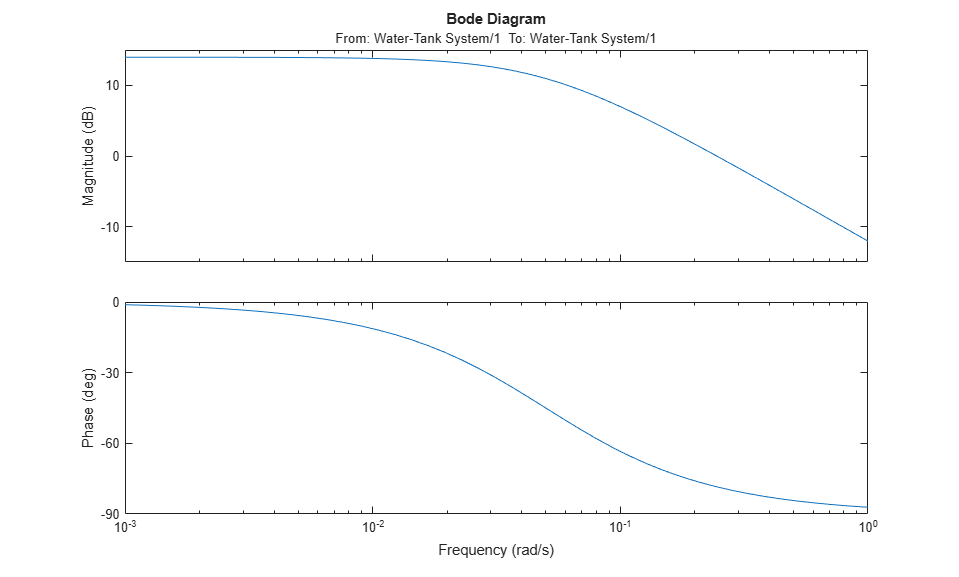## Linearize Plant

You can linearize a block or subsystem in your Simulink® model without defining separate analysis points for the block inputs and outputs. The software isolates the selected block from the rest of the model and computes a linear model of the block from the block inputs to the block outputs.

Linearizing a block in this way is equivalent to specifying open-loop input and open-loop output analysis points at the block inputs and outputs, respectively. For more information on specifying analysis points in your model, see Specify Portion of Model to Linearize.

### Linearize Plant Using Model Linearizer

This example shows how to linearize a plant subsystem in a Simulink model using Model Linearizer.

```mdl = 'watertank'; open_system(mdl)```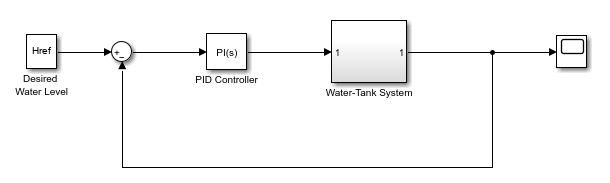For this model, the Water-Tank System block contains all the nonlinear dynamics. To linearize the block, use Model Linearizer.

To open Model Linearizer with the inputs and outputs of the block selected as the linearization I/O set, first open the Linearization tab. To do so, in the Simulink model window, in the Apps gallery, click Linearization Manager.

In the model, click the Water-Tank System block. Then, on the Linearization tab, click Linearize Block.

In Model Linearizer, on the Linear Analysis tab, in the Analysis I/Os drop-down list, the software sets the I/O set for linearization to `Block: Water-Tank System`.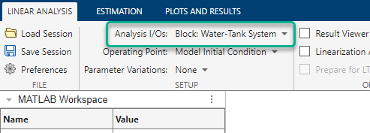Alternatively, if Model Linearizer is already open for your system, in the Simulink model window, click the Water-Tank System block. Then, in Model Linearizer, in the Analysis I/Os drop-down list, select `Linearize the Currently Selected Block`.

Tip

When the specified linearization I/O set is a block, you can highlight the block in the model by selecting the view option from the Analysis I/Os drop-down list. For example, to highlight the Water-Tank System block, select `View Water-Tank System`.

For this example, use the model operating point for linearization. The model operating point consists of the initial state values and input signals stored in the model. In Model Linearizer, on the Linear Analysis tab, in the Operating Point drop-down list, leave ```Model Initial Condition``` selected. For information on linearizing models at different operating points, see Linearize at Trimmed Operating Point and Linearize at Simulation Snapshot.

To linearize the specified block and generate a Bode plot for the resulting linear model, click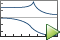Bode.

The software adds the linearized model, `linsys1`, to the Linear Analysis Workspace and generates a Bode plot for the model.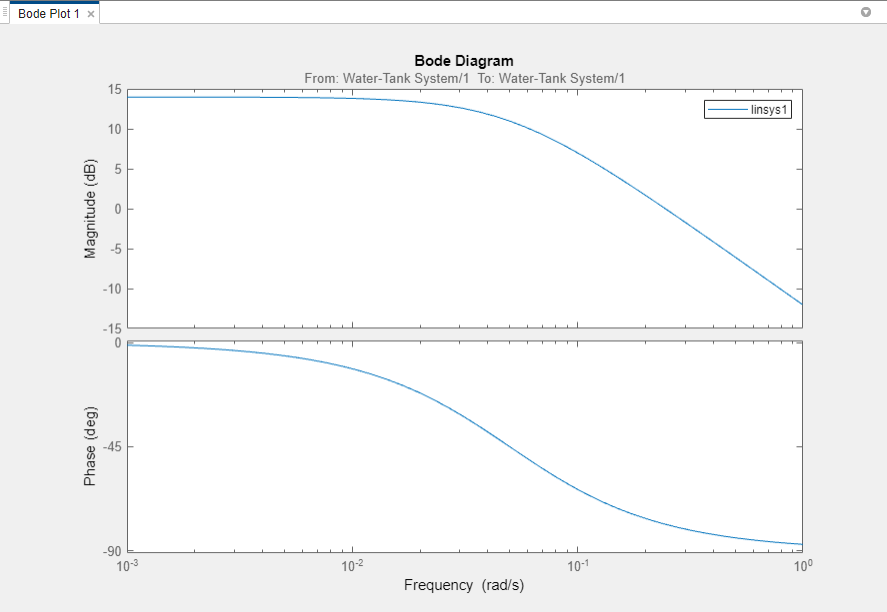For more information on analyzing linear models, see Analyze Results Using Model Linearizer Response Plots.

You can also export the linearized model to the MATLAB® workspace. To do so, in the data browser, in the Linear Analysis Workspace right-click `linsys1` and select Export to MATLAB Workspace.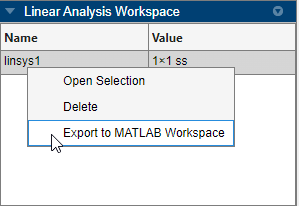### Linearize Plant at Command Line

This example shows how to linearize a plant subsystem in a Simulink® model using the `linearize` command.

```mdl = 'watertank'; open_system(mdl) ```For this system, the Water-Tank System block contains all the nonlinear dynamics. To linearize this subsystem, first specify its block path.

```blockpath = 'watertank/Water-Tank System'; ```

Then, linearize the plant subsystem at the model operating point.

```linsys1 = linearize(mdl,blockpath); ```

The model operating point consists of the initial state values and input signals stored in the model. For information on linearizing models at different operating points, see Linearize at Trimmed Operating Point and Linearize at Simulation Snapshot.

You can then analyze the response of the linearized model. For example, plot its Bode response.

```bode(linsys1) ```